Perl ProgrammingLearn about While Do-While and Until Loops in Perl

# Learn about While Do-While and Until Loops in Perl

Welcome to part two of the Loops topic. After discussing the first two types of Loops (for and foreach), we are going to continue with the remaining types.

while Loop
The while Loop uses a condition to control the iteration process. The loop continues to execute its body as long as the specified condition is met.

Syntax

```while (CONDITION)
{
Loop Body
}
```

Example
Let’s re-write the factorial example using the while Loop.

Consider the following script:

```#!/usr/bin/perl
#This script prompts the user to enter an integer
#and calculates its factorial using the while loop.
#Author: Eduonix
#Date: Nov 2015
\$result=1;
print ("Enter an Integer number: ");
\$a = <stdin>;
chomp \$a;
\$i = \$a;
while (\$i > 1)
{
\$result *= \$i;
\$i--;
}
print("Factorial of \$a equals \$result\n");
```

Will that do the job?! Let’s see: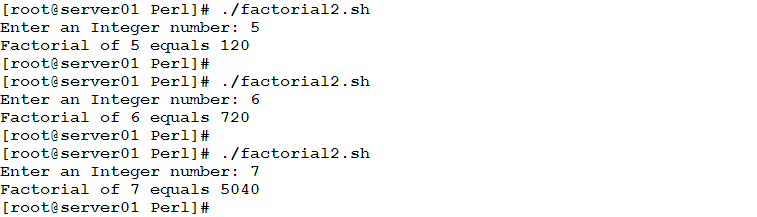For the while loop to achieve the same job that the for loop does, it needs to use a similar logic:

• A loop variable has been initialized:
`\$i = \$a;`
• A condition for the loop iteration is specified in the loop definition:
`while (\$i &gt; 1)`
• The loop variable is updated:
`\$i--;`

And also as in for loop, the while loop may not stop in either of the following cases:

• The logic of the condition is incorrect.
• You forget to update the loop variable.
• The loop variable is updated in the wrong way (for example, it is incremented instead of being decremented).

Now, let’s see how the while loop doing something that the for loop couldn’t do.

Example
We need to write a script that keeps asking the user to enter a number. This should continue as long as the user enters non-empty input. If the user enters an empty string, the loop exits. The script should print the total of the input numbers and their average.

To do so, check the following script:

```#!/usr/bin/perl
\$total = 0;
\$count = 0;
print("\nEnter a number: ");
while((\$i = <stdin>) != "")
{
chomp \$i;
\$total += \$i;
\$count++;
print("Enter a number: ");
}
print ("\nTotal = \$total\n");
print ("Average = ",\$total / \$count,"\n");
```

Let’s see it in action.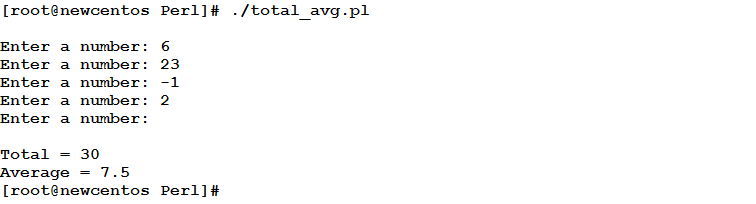As you see in the output, the script continues to ask the user to enter a number as long as the user enters something. When the user enters an empty string (by just pressing Enter), the loop exits, the total and average are calculated, and then printed to the user.

do…while Loop
The do…while loop is very similar to the while loop in its logic. Both types repeatedly execute the loop body as long as a specific condition is met. The loop exits when the condition becomes false. The only difference is that the condition is written after the loop body. That means the do…while loop will be always guaranteed to execute at least one iteration.

Syntax

```do
{
Loop Body
}
While (CONDITION);
```

Pay attention to the semi-colon after the condition.

Example
Let’s rewrite the previous example using the do…while loop.

```#!/usr/bin/perl
\$total = 0;
\$count = 0;
do{
chomp \$i;
\$total += \$i;
\$count++;
print("Enter a number: ");
}
while((\$i = <stdin>) != "");
\$count--;
print ("\nTotal = \$total\n");
print ("Average = ",\$total / \$count,"\n");
```

This modified version should give the same results as the previous one.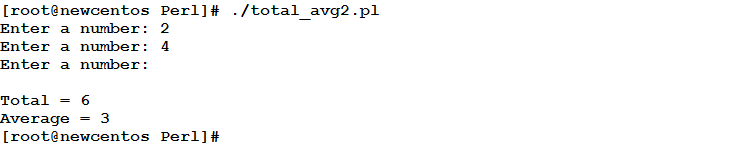Until Loop
Until this condition becomes true, continue to execute the loop body“. This is the logic of the until Loop.

Syntax

```Until (CONDITION)
{
Loop Body
}
```

In contrary to the while and do…while loops, the until loop continues to execute the loop body as long as its condition is not met (evaluates to false).

Example
We have written two versions of the script that calculates the total and average of input numbers using the while and do…while loops. Now, let’s see how to do it using the until loop.

```#!/usr/bin/perl
\$total = 0;
\$count = 0;
print("\nEnter a number: ");
until((\$i = <stdin>) == "")
{
chomp \$i;
\$total += \$i;
\$count++;
print("Enter a number: ");
}
print ("\nTotal = \$total\n");
if (\$count != 0)
{ print ("Average = ",\$total / \$count,"\n"); }
```

As you see, the condition used here is the opposite of the one used in the while and the do…while versions of the script.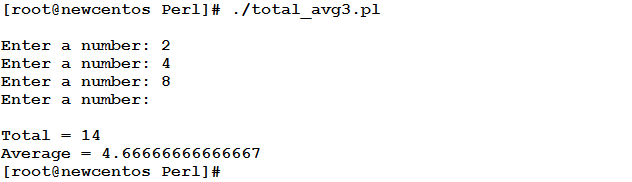Controlling Loops
There will be cases wherein you need to skip executing the remaining lines of the loop body in a loop iteration, or to exit the loop completely. Both cases should be controlled by a condition.

The next Statement
The next statement is used to skip the remaining lines in a loop iteration, and transfer the control back to top of the loop for the next iteration.

Emergency Exit (The last Statement)
The last statement terminates a loop. Once seen, the control is transferred to the next statement after loop’s terminating brace.

Example
We need to write a script that repeatedly prompts the user to enter a number, and tells whether this number is odd or even. If the user enters a zero, the script exits.

Consider the following code:

```while (true)
{
print ("Enter an integer number: ");
\$num = <stdin>;
last if (\$num == 0);
if (\$num % 2 ==0)
{
print ("\tEven number\n");
}
else
{
print ("\tOdd number\n");
}
}
```

When executed, it should give an output like the following: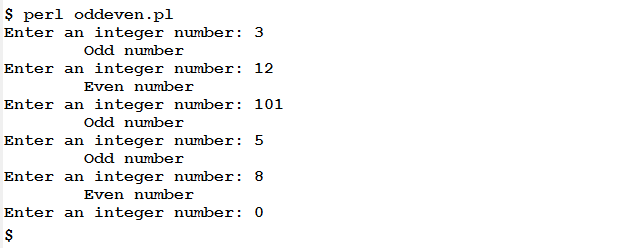Summary
In this article, we have continued with what we started in the article before.

• The while and do…while loops use similar logic. Both iterate as long as a specific condition is met. When the condition becomes false, the loop exits.
• The until loop iterates until as specific condition becomes true.
• Loops can be controlled to either skip executing an iteration, or to exit the loop completely. The decision to skip or exit a loop should be controlled by a condition.

That is it about loops. The next article gets us back to variables, with the second type of variables: Arrays.
See you there.

### Build A Front End Of an e-Commerce Site Using React

21,501Fans
4,213Followers
104,000Subscribers

### Build A Front End Of an e-Commerce Site Using React

- Advertisement -